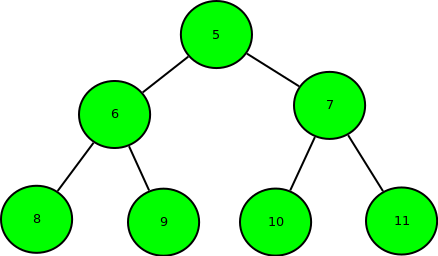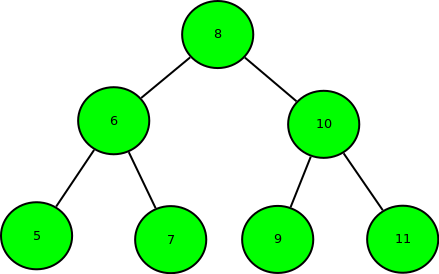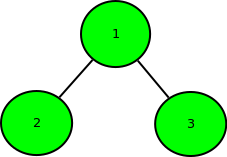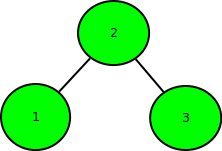Skip to content
Related Articles
Minimum swap required to convert binary tree to binary search tree
• Difficulty Level : Medium
• Last Updated : 12 May, 2021

Given the array representation of Complete Binary Tree i.e, if index i is the parent, index 2*i + 1 is the left child and index 2*i + 2 is the right child. The task is to find the minimum number of swap required to convert it into Binary Search Tree.

Examples:

```Input : arr[] = { 5, 6, 7, 8, 9, 10, 11 }
Output : 3
Binary tree of the given array:``````Swap 1: Swap node 8 with node 5.
Swap 2: Swap node 9 with node 10.
Swap 3: Swap node 10 with node 7.``````So, minimum 3 swaps are required.

Input : arr[] = { 1, 2, 3 }
Output : 1
Binary tree of the given array:````After swapping node 1 with node 2.``So, only 1 swap required.`

The idea is to use the fact that inorder traversal of Binary Search Tree is in increasing order of their value.
So, find the inorder traversal of the Binary Tree and store it in the array and try to sort the array. The minimum number of swap required to get the array sorted will be the answer. Please refer below post to find minimum number of swaps required to get the array sorted.
Minimum number of swaps required to sort an array
Time Complexity: O(n log n).

## C++

 `// C++ program for Minimum swap required``// to convert binary tree to binary search tree``#include``using` `namespace` `std;` `// Inorder Traversal of Binary Tree``void` `inorder(``int` `a[], std::vector<``int``> &v,``                        ``int` `n, ``int` `index)``{``    ``// if index is greater or equal to vector size``    ``if``(index >= n)``        ``return``;``    ``inorder(a, v, n, 2 * index + 1);``    ` `    ``// push elements in vector``    ``v.push_back(a[index]);``    ``inorder(a, v, n, 2 * index + 2);``}` `// Function to find minimum swaps to sort an array``int` `minSwaps(std::vector<``int``> &v)``{``    ``std::vector > t(v.size());``    ``int` `ans = 0;``    ``for``(``int` `i = 0; i < v.size(); i++)``        ``t[i].first = v[i], t[i].second = i;``    ` `    ``sort(t.begin(), t.end());``    ``for``(``int` `i = 0; i < t.size(); i++)``    ``{``        ``// second element is equal to i``        ``if``(i == t[i].second)``            ``continue``;``        ``else``        ``{``            ``// swaping of elements``            ``swap(t[i].first, t[t[i].second].first);``            ``swap(t[i].second, t[t[i].second].second);``        ``}``        ` `        ``// Second is not equal to i``        ``if``(i != t[i].second)``            ``--i;``        ``ans++;``    ``}``    ``return` `ans;``}` `// Driver code``int` `main()``{``    ``int` `a[] = { 5, 6, 7, 8, 9, 10, 11 };``    ``int` `n = ``sizeof``(a) / ``sizeof``(a);``    ``std::vector<``int``> v;``    ``inorder(a, v, n, 0);``    ``cout << minSwaps(v) << endl;``}` `// This code is contributed by code_freak`

## Python3

 `# Python3 program for Minimum swap required``# to convert binary tree to binary search tree` `# Inorder Traversal of Binary Tree``def` `inorder(a, n, index):``    ` `    ``global` `v``    ` `    ``# If index is greater or equal to``    ``# vector size``    ``if` `(index >``=` `n):``        ``return``    ` `    ``inorder(a, n, ``2` `*` `index ``+` `1``)` `    ``# Push elements in vector``    ``v.append(a[index])``    ``inorder(a, n, ``2` `*` `index ``+` `2``)` `# Function to find minimum swaps``# to sort an array``def` `minSwaps():``    ` `    ``global` `v``    ``t ``=` `[[``0``, ``0``] ``for` `i ``in` `range``(``len``(v))]``    ``ans ``=` `-``2` `    ``for` `i ``in` `range``(``len``(v)):``        ``t[i][``0``], t[i][``1``] ``=` `v[i], i` `    ``t, i ``=` `sorted``(t), ``0` `    ``while` `i < ``len``(t):``        ` `        ``# break``        ``# second element is equal to i``        ``if` `(i ``=``=` `t[i][``1``]):``            ``i ``+``=` `1``            ``continue``        ``else``:``            ` `            ``# Swaping of elements``            ``t[i][``0``], t[t[i][``1``]][``0``] ``=` `t[t[i][``1``]][``0``], t[i][``0``]``            ``t[i][``1``], t[t[i][``1``]][``1``] ``=` `t[t[i][``1``]][``1``], t[i][``1``]` `        ``# Second is not equal to i``        ``if` `(i ``=``=` `t[i][``1``]):``            ``i ``-``=` `1` `        ``i ``+``=` `1` `        ``ans ``+``=` `1` `    ``return` `ans` `# Driver Code``if` `__name__ ``=``=` `'__main__'``:``    ` `    ``v ``=` `[]``    ``a ``=` `[ ``5``, ``6``, ``7``, ``8``, ``9``, ``10``, ``11` `]``    ``n ``=` `len``(a)``    ``inorder(a, n, ``0``)` `    ``print``(minSwaps())` `# This code is contributed by mohit kumar 29`

## Javascript

 ``

Output:

`3`

Exercise: Can we extend this to normal binary tree, i.e., a binary tree represented using left and right pointers, and not necessarily complete?

This article is contributed by Anuj Chauhan. If you like GeeksforGeeks and would like to contribute, you can also write an article using write.geeksforgeeks.org or mail your article to contribute@geeksforgeeks.org. See your article appearing on the GeeksforGeeks main page and help other Geeks.
Please write comments if you find anything incorrect, or you want to share more information about the topic discussed above.

Attention reader! Don’t stop learning now. Get hold of all the important DSA concepts with the DSA Self Paced Course at a student-friendly price and become industry ready.  To complete your preparation from learning a language to DS Algo and many more,  please refer Complete Interview Preparation Course.

In case you wish to attend live classes with industry experts, please refer DSA Live Classes

My Personal Notes arrow_drop_up